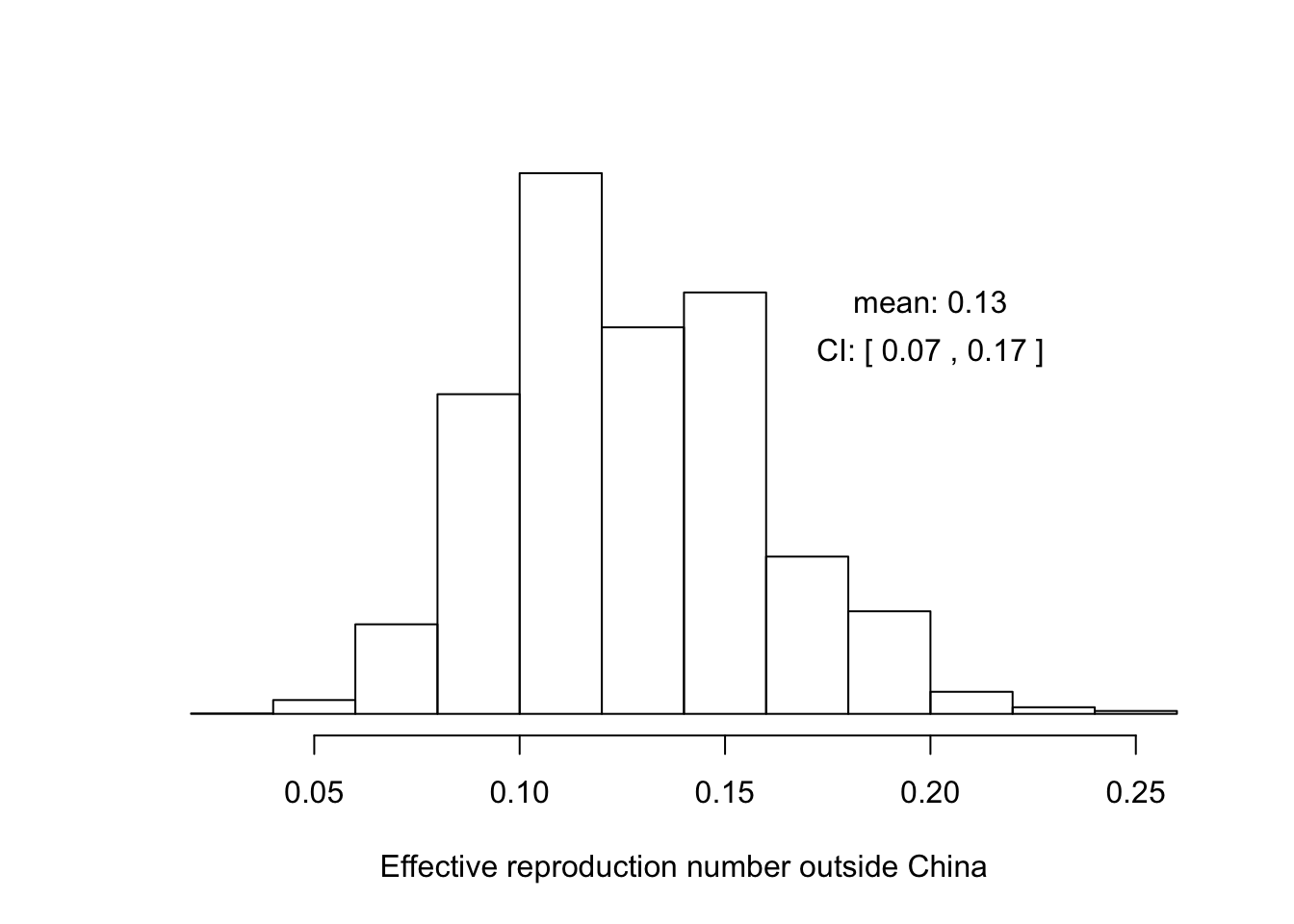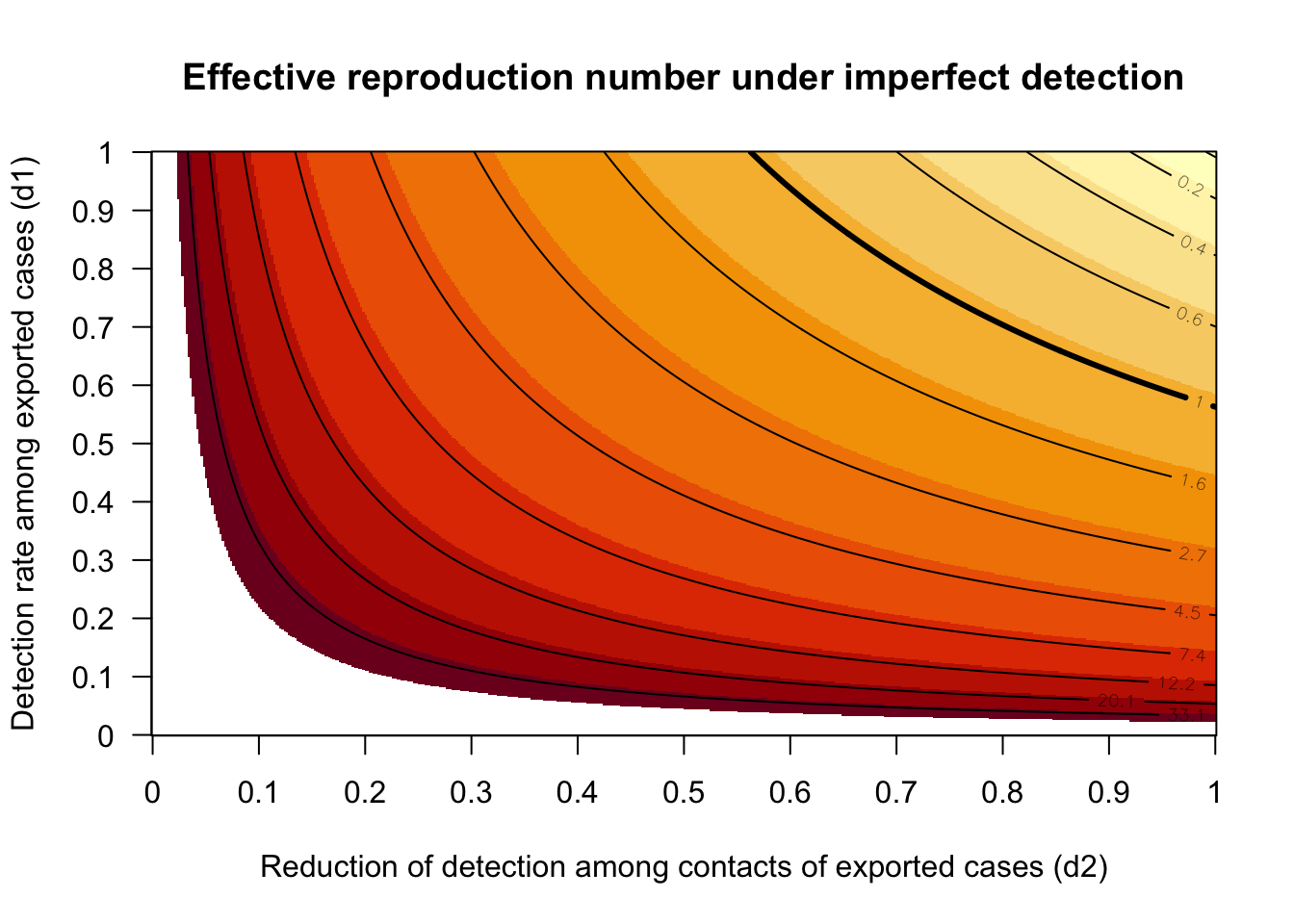### What is the average $$R_{\text{eff}}$$ outside of China?

At this writing (16 February 2020) a number of cases of COVID-19 have been exported outside of China. Some of these have led to secondary transmission, while others have not. Some important questions include

1. Is the current level of vigilance adequate for detection and containment?
2. What potential is there for sustained transmission in these settings?

However, because there is relatively little data from secondary transmission chains, it is not possible to estimate the effective reproduction number, $$R_{\text{eff}}$$, in these settings directly. Nonetheless, it seems reasonable to think that the relative number of secondary transmission events contains information about the effective reproduction number in these settings. The following is a calculation to estimate the average effective reproduction number of cases exported outside China.

### Calculation for perfect observation

During the early phases of an $$SIR$$ epidemic, the increase in the number of infected cases may be approximated by a birth-death process where the birth rate is given by the transmissibility $$\beta$$ and the death rate is given by the recovery rate $$\gamma$$. It is understood from context that both $$\beta$$ and $$\gamma$$ are considered at their effective values and may be different from the natural transmissibility and recovery rates due to social distancing, quarantine, active case identification and isolation, etc.

A case exported to a susceptible population may give rise to secondary infections or may be isolated before such infections occur. The probability $$p_0$$ that no secondary infection occurs is given by the proportional propensity $$p_0=\frac{\gamma}{\beta+\gamma}$$. Taking the reciprocal of each side of this equation, we have

$\begin{equation} \frac{1}{p_0} = \frac{\beta+\gamma}{\gamma} = \frac{\beta}{\gamma} + \frac{\gamma}{\gamma} = R_{\text{eff}} + 1 \end{equation}$

A simple rearrangement yields

$\begin{equation} R_{\text{eff}} = \frac{1}{p_0}-1. \end{equation}$

The probability $$p_0$$ may be estimated from the fraction of exported cases that have not led to secondary transmission leading to

$\begin{equation} \label{eq:estimator} R_{\text{eff}} = \frac{X}{Y}-1 \end{equation}$

where $$X$$ is the total number of exported cases and $$Y$$ is the number of exported cases without secondary transmission. Note that $$X$$ and $$Y$$ are both observed.

The WHO Situation Report for 13 February 2020 reported: “As of 10am CET 13 Feb 2020, a total of 170 cases of COVID-19 who had a travel history to China have been reported outside of China. The vast majority of these (151, 89%) do not appear to lead to further transmission of the virus, while the remaining 19 have been associated with onward transmission within 12 distinct groups of epidemiologically linked cases.”"

Thus, we estimate that the realized effective reproduction number outside China during the period of these reports was $$R_{\text{eff}} = \frac{170}{151} -1 = 0.1258278...$$. By resampling from these observations with replacement, we estimate the uncertainty around this estimate with a confidence interval of [0.07, 0.17].Assumptions of this calculation include:

1. That all exported transmission chains have been observed, i.e. that there are no significant ongoing, undetected chains of transmission elsewhere in the world.
2. This estimate for $$R_{\text{eff}}$$ is only valid for the set of locations for which exported cases are reported. Particularly, places that differ substantially with respect to surveillance capacity, vigilance, and ability to isolate will be more vulnerable than this calculation suggests. Moreover, we assume that $$\beta$$ and $$\gamma$$ are similar in all reporting locations.
3. That all chains determined to have ended with no secondary transmission are correctly classified.

### Calculation with imperfect observation

The first and third assumptions can be investigated further. Recall that equation has the form

$\begin{equation} \label{eq:general} R_{\text{eff}} = \frac{\text{total exported cases}}{\text{exported cases without secondary transmission}}-1. \end{equation}$

Next, we allow that neither exported cases nor the secondary chains of transmission that they generate may be perfectly observed. That is, we distinguish detected from undetected exported cases and detected from undetected cases among contacts of exported cases. We denote the fraction of exported cases that are detected by $$0 \leq d_1 \leq 1$$ so $$d_1$$ is the detection rate of exported cases. We assume that cases among contacts of exported cases are even less likely to be detected than the exported cases themselves. This seems reasonable under current surveillance strategies that closely observe travelers from regions with active transmission. Thus, we denote the reduction in detection of transmission chains among contacts of exported cases by a factor $$0 \leq d_2 \leq 1$$ so that the detection rate among contacts of exports is $$d_1 \times d_2$$.

Equation should now be written in terms of the observables $$X=170$$ and $$Y=151$$ in addition to unobserved quantities $$U_1$$ (the number of undetected exported cases), $$U_2$$ (detected exported cases with undetected chains of secondary transmission), and $$U_3$$ (undetected exports with no undetected chains of transmission). Now we have

$\begin{equation} R_{\text{eff}} = \frac{X+U_1}{Y - U_2 + U_3}-1. \end{equation}$

Formulas for $$U_1$$ and $$U_2$$ in terms of $$X$$, $$Y$$, $$d_1$$ and $$d_2$$ are

$\begin{equation} \label{eq:u1} U_1 = \left ( \frac{1-d_1}{d_1}\right) \times X \end{equation}$

and

$\begin{equation} \label{eq:u2} U_2 = (1-{d_1d_2}) \times Y. \end{equation}$

A formula for $$U_3$$ comes from multiplying the total number of undetected exports, $$U_1$$, by the probability $$p_0$$ of not generating any secondary chains of transmission.

$\begin{equation} \label{eq:u3} U_3 = X \times \left ( \frac{1-d_1}{d_1}\right) \times p_0. \end{equation}$

Writing equation in terms of $$R_{\text{eff}}$$ instead of $$p_0$$ we have

$\begin{equation} \label{eq:u3-2} U_3 = X \times \left ( \frac{1-d_1}{d_1}\right) \times \left (\frac{1}{R_{\text{eff}}+1} \right ). \end{equation}$

Putting it all together we have the equation

$\begin{equation} R_{\text{eff}} = \frac{X+\left ( \frac{1-d_1}{d_1}\right) X}{Y - (1-{d_1d_2}) Y + X \left ( \frac{1-d_1}{d_1}\right) \left (\frac{1}{R_{\text{eff}}+1} \right )}-1. \end{equation}$

$$R_{\text{eff}}$$ may be found numerically by solving for the root of $$g = f - R_{\text{eff}}$$.

Here we test the function assuming perfect observation by setting $$d_1 = 1$$ and $$d_2=1$$. In this limit case, the numerical solution agrees with the exact solution to at least seven decimal places.

## $root ##  0.1258278 ## ##$f.root
##  0
##
## $iter ##  1 ## ##$init.it
##  NA
##
## \$estim.prec
##  499.8742

Now, we solve over a range of $$d_1$$ and $$d_2$$. Clearly, the failure to detect exported cases or cases among contacts of exported cases yields a biased estimate of $$R_{\text{eff}}$$. The heavy solid line indicates the critical boundary at $$R_{\text{eff}}=0$$. Given that there have not been a significant number of cases identified without a plausible exposure scenario (e.g. travel history to China or exposure to a known case), it seems reasonable to assume that $$d_1$$ is relatively close to 1. It is hoped that $$d_2$$ has also remained close to 1, for instance through effective contract tracing. Assuming $$d_1=d_2=0.9$$, this estimator yields an average $$R_{\text{eff}}$$ outside of China of 0.39.It is strongly suspected that COVID-19 transmission is hetereogeneous, such that a small fraction of infections contribute disproportionately to onward transmission. The consequences of this heterogeneity can be investigated by modeling the secondary infection rate as negative binomial random variable. Using this distribution, the probability an imported case fails to transmit is

$\begin{equation} \label{eq:ng} p_0 = (1 + R_{\text{eff}}/k)^{-k}, \end{equation}$

where $$k$$ is a describing the effect of heterogeneity. The model presented in the first two sections of this report is a special case where $$k=1$$. The value of $$k$$ for COVID-19 in the United States has not yet been estimated. Here, we investigate two different values for $$k$$.

1. Following Blumberg et al. (https://www.medrxiv.org/content/10.1101/2020.02.08.20021311v1), we assume $$k=0.3$$, which is described as “a high degree of disease heterogeneity”.
2. Following Lloyd-Smith et al. (https://www.nature.com/articles/nature04153), we use the value $$k=0.16$$, which is estimated for the dispersion of SARS transmission in Singapore.

For transmission following a negative binomial distrbution

$\begin{equation} p_0 = \left( 1+\frac{R_{\text{eff}}}{k}\right)^{-k} \end{equation}$

which may be rearranged to

$\begin{equation} R_{\text{eff}} = k \left(\frac{1}{p_0} \right)^{\frac{1}{k}} - k \end{equation}$

For transmission following a negative binomial distribution, $$R_{\text{eff}}$$ may be obtained from data with imperfect detection using the formula

$\begin{equation} R_{\text{eff}} = k \left( \frac{X+\left ( \frac{1-d_1}{d_1}\right) X}{Y - (1-{d_1d_2}) Y + X \left ( \frac{1-d_1}{d_1}\right) \left (\frac{1}{(R_{\text{eff}}/k+1)^k} \right )} \right)^{\frac{1}{k}}-k. \end{equation}$